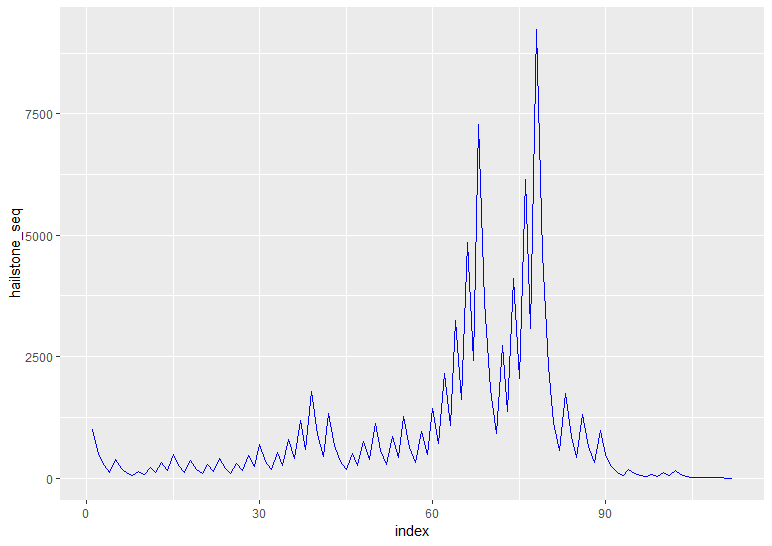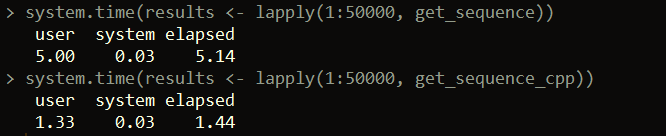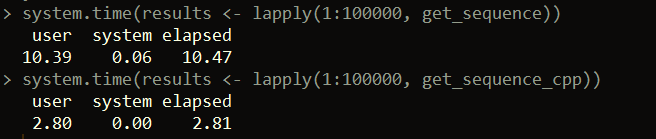Want to share your content on R-bloggers? click here if you have a blog, or here if you don't.## Background

The Collatz Conjecture is a famous unsolved problem in number theory. If you’re not familiar with it – the conjecture is very simple to understand, yet, no one has been able to mathematically prove that the conjecture is true (though it’s been shown to be true for an enormous number of cases).

The conjecture states the following:

Start with any whole number. If the number is even, divide by two. If the number is odd, multiply the number by three and add one. Then, repeat this logic with the result number. Eventually you’ll end up with the number one. Effectively, this is can written like this:

```For any whole number n:

If n mod 2 == 0, then n = n / 2

Else n = 3 * n + 1

Repeat until n = 1

```

The conjecture states that this is true for any positive whole number n.

Here’s a couple sequence examples:

```n = 15

15, 46, 23, 40, 20, 10, 5, 16, 8, 4, 2, 1

n = 7

7, 22, 11, 34, 17, 52, 26, 13, 40, 20, 10, 5, 16, 8, 4, 2, 1

```

This post starts by showing how to generate a number’s result sequence (sometimes called “hailstone sequence”) in R, along with how to produce corresponding visuals. Then we’ll show how we can speed up testing the conjecture using Rcpp.

## Generating a number’s sequence with R

We can break the code we need to write into two functions. The first will just take a whole number as input and return the next number in sequence, based upon the logic we outlined above. If num = 1, we’ll just return NULL because we end the sequence at 1.

```
next_num <- function(num)
{
if(num == 1)
return(NULL)

if(num %% 2 == 0)
return(num / 2)

return(3 * num + 1)

}

```

Next, we just need to write code that will call this function repeatedly until the number one is returned. Each time we call next_num, we append it to the end of the vector result. At the end, we return the sequence of numbers in the journey from the initial input to one.

```get_sequence <- function(input)
{

result <- input
while(input != 1)
{
input <- next_num(input)
result <- c(result, input)

}

return(result)
}

```

Here’s some examples of calling our function:## Plotting the hailstone sequence

We can create a line plot of the hailstone sequence result for an input like below using the ggplot2 package.

```library(ggplot2)

plot_sequence <- function(input)
{
hailstone_seq <- get_sequence(input)

hailstone_df <- data.frame(hailstone_seq = hailstone_seq, index = 1:length(hailstone_seq))

ggplot(data=hailstone_df, aes(x = index, y = hailstone_seq, group = 1)) +
geom_line(color = "blue")

}

```

For input = 1000:For input = 10,000## Testing the Collatz Conjecture with Rcpp

Now, what if we want to test the conjecture on a larger amount of numbers? We could use our R code above, but another way is to use Rcpp to re-write our functions. In this method, we compile C++ functions to perform our task into R functions. This allows us to create faster-running functions because of the efficiency of C++. If you haven’t used Rcpp before, I suggest you check out this for additional resources.

In the first part of our code, our function next_num_cpp looks very similar to our R function next_num. They are performing the same task, and the syntax is actually not so different in this case. More on the second function follows below the code.

```
// [[Rcpp::export]]
int next_num_cpp(int num) {
if(num == 1)
return num;

if(num % 2 == 0)
return num / 2;

return 3 * num + 1;

}

// [[Rcpp::export]]
IntegerVector get_sequence_cpp(int input) {

IntegerVector result;

result = input;

while(input > 1)
{
input = next_num_cpp(input);
result.insert(result.length(), input);

}

return result;

}

```

The second function, get_sequence_cpp, performs the same task as get_sequence. This time the syntax is a little different. Here we have to declare variable data types. To return a vector of integers, we use the IntegerVector data type. We start by setting the 0th element (C++ is zero-indexed) to the initial input. Then we start the loop. In the loop we generate the next number in the sequence (using next_num_cpp). This number is then appended to the end of the vector (using result.insert).

Now, let’s test how fast the Rcpp implementation works vs. the base R version.As you can see, there’s using Rcpp is several times faster than using base R to validate the conjecture. From the example above, in under three seconds, we’re able to validate the conjecture holds for the first 100,000 whole numbers. If we want to speed up testing the conjecture even more for larger ranges of numbers, we could also consider using parallelization (see this post for a similar example).